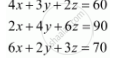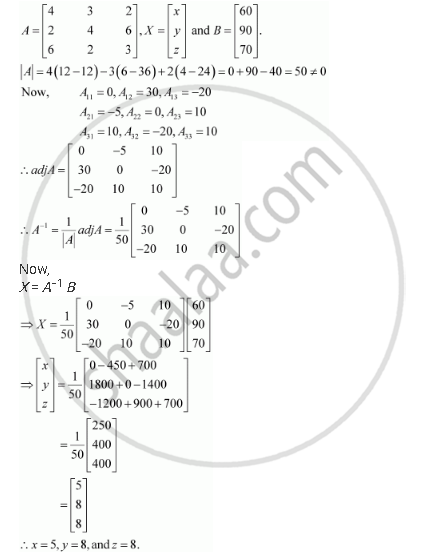Share

# The Cost of 4 Kg Onion, 3 Kg Wheat and 2 Kg Rice is Rs 60. the Cost of 2 Kg Onion, 4 Kg Wheat and 6 Kg Rice is Rs 90. the Cost of 6 Kg Onion 2 Kg Wheat and 3 Kg Rice is Rs 70. Find Cost of Each Item per Kg by Matrix Method. - CBSE (Science) Class 12 - Mathematics

ConceptApplications of Determinants and Matrices

#### Question

The cost of 4 kg onion, 3 kg wheat and 2 kg rice is Rs 60. The cost of 2 kg onion, 4 kg wheat and 6 kg rice is Rs 90. The cost of 6 kg onion 2 kg wheat and 3 kg rice is Rs 70. Find cost of each item per kg by matrix method.

#### Solution

Let the cost of onions, wheat, and rice per kg be Rs x, Rs y,and Rs z respectively.

Then, the given situation can be represented by a system of equations as:This system of equations can be written in the form of AX = B, whereHence, the cost of onions is Rs 5 per kg, the cost of wheat is Rs 8 per kg, and the cost of rice is Rs 8 per kg.

Is there an error in this question or solution?

#### Video TutorialsVIEW ALL 

Solution The Cost of 4 Kg Onion, 3 Kg Wheat and 2 Kg Rice is Rs 60. the Cost of 2 Kg Onion, 4 Kg Wheat and 6 Kg Rice is Rs 90. the Cost of 6 Kg Onion 2 Kg Wheat and 3 Kg Rice is Rs 70. Find Cost of Each Item per Kg by Matrix Method. Concept: Applications of Determinants and Matrices.
S